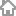-----  人品   师品   文品-----`百学须先立志。(朱熹) `
` `
`宝剑锋从磨砺出,梅花香自苦寒来。 `
` `
`笔落惊风雨,诗成泣鬼神。(杜甫) `
` `
`别裁伪体亲风雅,转益多师是汝师。(杜甫) `
` `
`博观而约取,厚积而薄发。(苏轼) `
` `
`博学之,审问之,慎思之,明辨之,笃行之。(《礼记》) `
` `
`不登高山,不知天之高也;不临深溪,不知地之厚也。(《荀子》) `
` `
`不飞则已,一飞冲天;不鸣则已,一鸣惊人。(司马迁) `
` `
`不患人之不己知,患不知人也。(孔子) `
` `
`采得百花成蜜后,为谁辛苦为谁甜。(罗隐) `
` `
`仓廪实则知礼节,衣食足则知荣辱。(《管子》) `
` `
`操千曲而后晓声,观千剑而后识器。(刘勰) `
` `
`察己则可以知人,察今则可以知古。(《吕氏春秋》) `
` `
`己所不欲,勿施于人。(论语) `
` `
`兼听则明,偏信则暗。(《资治通鉴》) `
` `
`见兔而顾犬,未为晚也;亡羊而补牢,未为迟也。(《战国策》) `
` `
`见义不为,非勇也。(论语) `
` `
`江山代有才人出,各领风骚数百年。(赵翼) `
` `
`金玉其外,败絮其中。(刘基) `
` `
`信言不美,美言不信。善者不辩,辩者不善。(老子) `